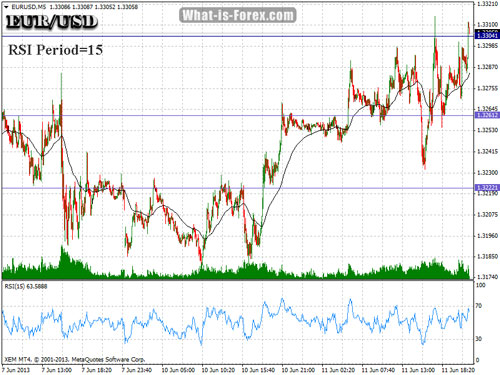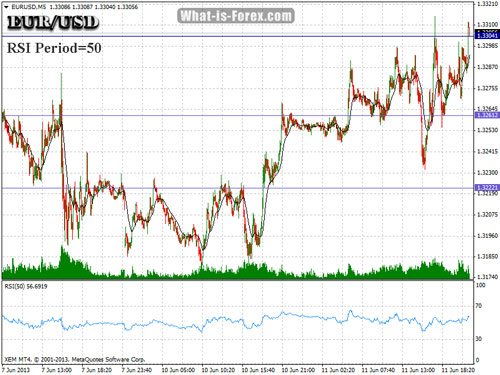What is the Relative Strength Index or RSI?

Relative Strength Index (RSI) is a very popular technical analysis indicator (oscillator) which aims to identify overbought and oversold market conditions of any traded asset. RSI is used in Forex, Stocks, and in other forms of online trading. RSI is scaled from 0 to 100. Generally speaking, the RSI level of positive 70, or more, indicates overbought market conditions, while the RSI level of negative 30 indicates oversold market conditions.

■ RSI 70+ Overbought

■ RSI 30- Oversold

• Identify overbought/oversold market levels (main use)
• Recognize potential historical support and resistance levels
• Evaluate the sustainability of the trend
• Early-spot reversals via crosses and slope divergences
• Optimize entries in the market

How can we calculate RSI?

Nowadays, the RSI indicator is embedded in almost every trading platform that provides technical analysis. RSI may be calculated using the following formula:

100

RSI = 100 - --------------

1 + RS*

*Where RS for the time period (t) can be calculated if we divide the Average of upward closings during the period (t) to the Average of downward closings during the same period (t)

Using RSI to Confirming the Price Trend

The readings of RSI may be used for confirming new trends. In general, an upward trend must have an RSI reading above 50 while a downward trend must have an RSI reading below 50.

■ RSI 50+ Possible Upward Trend Confirmation

■ RSI 50- Possible Downward Trend Confirmation

Note: If you are a day-trader then use the RSI indicator only in the 5M chart. If the market is ranging and you trade long at RSI=30 and trade short at RSI=70 you can make very good profits. Remember to use the RSI at the 5M chart.

The Relative Strength Index oscillates between zero (0) and one hundred (100):

• An asset is considered overbought when RSI is above 70
• An asset is considered oversold when RSI is below 30
• When trading high-volatility assets it is recommended to focus on 80,20 instead of 70,30.

Experimenting with RSI

RSI as another technical analysis indicator may be adjusted to a preferable time frame. The fewer periods used the more aggressive RSI indicates an asset while the more periods used the slower the indicator becomes. The standard RSI period value is 14.

Note:

• The standard periods for RSI are 14, however, depending on the volatility of the underlying asset you can modify the number of periods in order to enhance accuracy.
• In the following two charts you may compare RSI period=15 and RSI period=50.

Chart: RSI period 15 on EURUSDChart: RSI period 50 on EURUSDCOMPARE: » | » Forex Bonus

◘ What is RSI?

What-is-Forex.com